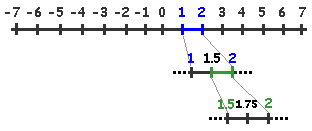Home    |    Teacher    |    Parents    |    Glossary    |    About UsIn this lesson we look at some properties that apply to all real numbers. If you learn these properties, they will help you solve problems in algebra. Let's look at each property in detail, and apply it to an algebraic expression.

#1. Commutative properties
The commutative property of addition says that we can add numbers in any order. The commutative property of multiplication is very similar. It says that we can multiply numbers in any order we want without changing the result.

5a + 4 = 4 + 5a

multiplication
3
x 8 x 5b = 5b x 3 x 8

#2. Associative properties
Both addition and multiplication can actually be done with two numbers at a time. So if there are more numbers in the expression, how do we decide which two to "associate" first? The associative property of addition tells us that we can group numbers in a sum in any way we want and still get the same answer. The associative property of multiplication tells us that we can group numbers in a product in any way we want and still get the same answer.

(4x + 2x) + 7x = 4x + (2x + 7x)

multiplication
2x2(3y) = 3y(2x2)

#3. Distributive property
The distributive property comes into play when an expression involves both addition and multiplication. A longer name for it is, "the distributive property of multiplication over addition." It tells us that if a term is multiplied by terms in parenthesis, we need to "distribute" the multiplication over all the terms inside.

2x(5 + y) = 10x + 2xy

Even though order of operations says that you must add the terms inside the parenthesis first, the distributive property allows you to simplify the expression by multiplying every term inside the parenthesis by the multiplier. This simplifies the expression.

#4. Density property
The density property tells us that we can always find another real number that lies between any two real numbers. For example, between 5.61 and 5.62, there is 5.611, 5.612, 5.613 and so forth.

Between 5.612 and 5.613, there is 5.6121, 5.6122 ... and an endless list of other numbers!#5. Identity property
The identity property for addition tells us that zero added to any number is the number itself. Zero is called the "additive identity." The identity property for multiplication tells us that the number 1 multiplied times any number gives the number itself. The number 1 is called the "multiplicative identity."

 Homework Help | Algebra | The Basics of AlgebraEmail this page to a friendSearch·  Properties of     Real Numbers·  Exponents·  Evaluating expressions·  Like terms·  SimplifyingFirst Glance In Depth Examples WorkoutProperties of real numbers